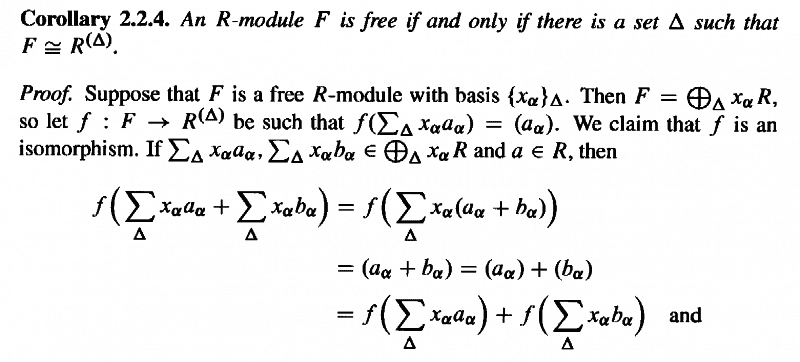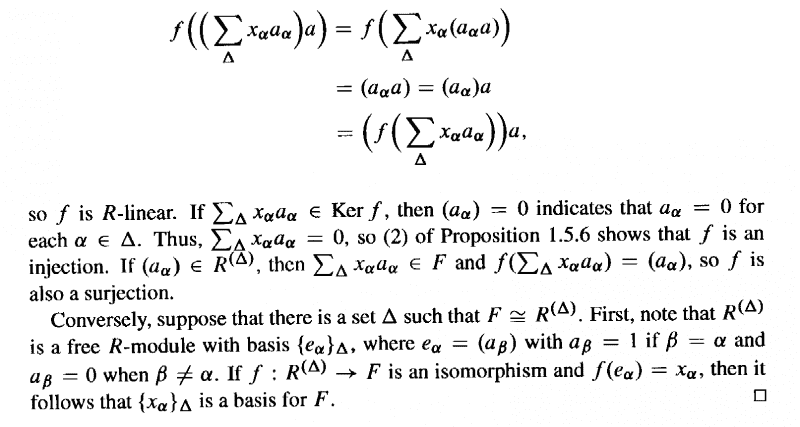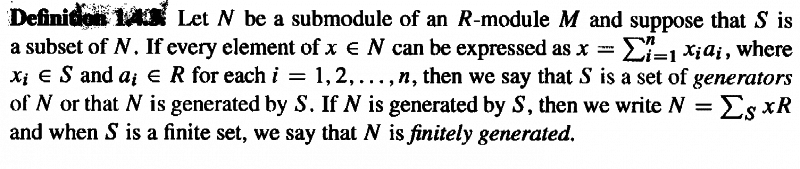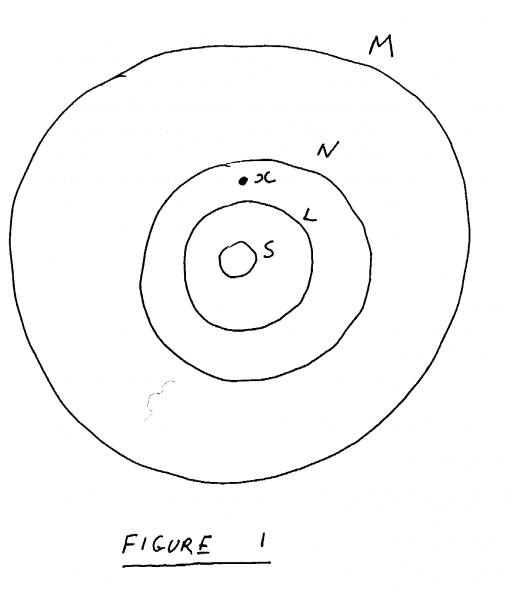# Free Modules - Bland Corollary 2.2.4 - Issue regarding finite generation of modules

Gold Member
I am reading Paul E. Bland's book, "Rings and Their Modules".

I am trying to understand Section 2.2 on free modules and need help with the proof of Corollary 2.2.4.

Corollary 2.2.4 and its proof read as follows:In the second last paragraph of Bland's proof above we read:

" ... ... If $(a_\alpha) \in R^{ ( \Delta ) }$, then $\sum_\Delta x_\alpha a_\alpha \in F$ ... ... "

My question is as follows:

How, exactly, do we know that $(a_\alpha) \in R^{ ( \Delta ) }$ implies that $\sum_\Delta x_\alpha a_\alpha \in F$ ... ... that is, is it possible that for some $(a_\alpha) \in R^{ ( \Delta ) }$ there is no element $x$ such that $x = \sum_\Delta x_\alpha a_\alpha \in F$?

To make sure my question is clear ... ...

If F is a free R-module with basis $\{ x_\alpha \}_\Delta$, then every element $x \in F$ can be expressed (generated) as a sum of the form:

$x = \sum_\Delta x_\alpha a_\alpha$

... ... BUT ... ... does this mean that for any element $(a_\alpha) \in R^{ ( \Delta ) }$ there is actually an element $x \in F$ such that $x = \sum_\Delta x_\alpha a_\alpha$?

... OR ... to put it another way ... could it be that for some element $(a_\alpha) \in R^{ ( \Delta ) }$ there is actually NO element $x \in F$ such that $x = \sum_\Delta x_\alpha a_\alpha$?

Can someone please clarify this issue for me?

Peter

***NOTE***

I thought I would try to clarify just exactly why I am perplexed about the nature of the generation of a module or submodule by a set $S$.

Bland defines the generation of a submodule of $N$ of an $R$-module $M$ as follows:Now consider a submodule $L$ of $M$ such that $L \subset N$.

See Figure $1$ as follows:Now $L$, like $N$, will (according to Bland's definition) also be generated by $S$, since every element $y \in L$ will be able to be expressed as a sum

$y = \sum_{\Delta} x_\alpha a_\alpha$

where $x_\alpha \in S$ and $a_\alpha \in R$

This is possible since every element of $N$ (and hence $L$) can be expressed this way.

However ... ... if we consider $x \in N$ such that $x \notin L$ then

$x = \sum_{\Delta} x_\alpha a_\alpha$

for some $x_\alpha, a_\alpha$

... ... BUT ... ... in this case, there is no $(a_\alpha) \in R^{ ( \Delta ) }$ such that

$\sum_{\Delta} x_\alpha a_\alpha \in L$

... ... BUT ... ... this is what is assumed in Bland's proof of Corollary $2.2.4$???

Can someone please clarify this issue ...

Peter

#### Attachments

Thanks for the post! This is an automated courtesy bump. Sorry you aren't generating responses at the moment. Do you have any further information, come to any new conclusions or is it possible to reword the post?

mathwonk
Homework Helper
2020 Award
"If F is a free R-module with basis {xα}Δ, then every element x∈F can be expressed (generated) as a sum of the form:

x=∑Δxαaα

... ... BUT ... ... does this mean that for any element (aα)∈R(Δ) there is actually an element x∈F such that x=∑Δxαaα?